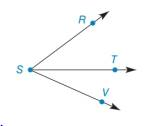Chapter 1.4, Problem 16E### Elementary Geometry for College St...

6th Edition
Daniel C. Alexander + 1 other
ISBN: 9781285195698

#### Solutions

Chapter
Section### Elementary Geometry for College St...

6th Edition
Daniel C. Alexander + 1 other
ISBN: 9781285195698
Textbook Problem
31 views

# Given: m ∠ R S T = 39 ° m ∠ T S V = 23 ° Find: m ∠ R S VExercises 16 − 24

To determine

To find:

The measure of angle RSV.

Explanation

Given:

mRST=39°mTSV=23°

The given figure is,

Definition:

If a point say M lies in the interior of PQR, then mPQM+mRQM=mPQR.

Calculation:

Consider the figure,

T is the interior point of the angle RSV

### Still sussing out bartleby?

Check out a sample textbook solution.

See a sample solution

#### The Solution to Your Study Problems

Bartleby provides explanations to thousands of textbook problems written by our experts, many with advanced degrees!

Get Started

#### In Exercises 2336, find the domain of the function. 32. f(x)=1x2+x2

Applied Calculus for the Managerial, Life, and Social Sciences: A Brief Approach

#### The quadratic approximation for at a = 1 is:

Study Guide for Stewart's Single Variable Calculus: Early Transcendentals, 8th

#### Area In Exercises 89-92, find the area of the given region. y=5xx4+1

Calculus: Early Transcendental Functions (MindTap Course List)